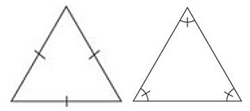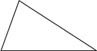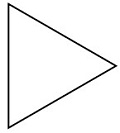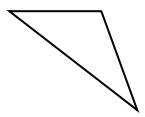# Classifying scalene, isosceles, and equilateral triangles by side lengths or angles

#### Complete Python Prime Pack

9 Courses     2 eBooks

#### Artificial Intelligence & Machine Learning Prime Pack

6 Courses     1 eBooks

#### Java Prime Pack

9 Courses     2 eBooks

Triangles can be classified according to their sides or according to their angles. All of each side or angle may be of different or the same sizes; any two sides or angles may be of the same size.

The types of triangles classified by their sides are the following.

Equilateral triangle − A triangle with all three sides equal in length is an equilateral triangle. All angles of an equilateral triangle are equal too.

The slash marks indicate equal measure.Isosceles triangle − A triangle in which at least two sides are of equal length is an isosceles triangle.

An isosceles triangle also has two of its angles equal in measure.Scalene triangle − A triangle with all three sides of different lengths is a scalene triangle. The angles of a scalene triangle are also of different measure.Identify the given triangle as an equilateral, isosceles or a scalene triangle.### Solution

Step 1:

Given triangle has all sides of same length.

Step 2:

So given triangle is an equilateral triangle.

Identify the given triangle as an equilateral, isosceles or a scalene triangle.### Solution

Step 1:

Given triangle has sides of different lengths.

Step 2:

So given triangle is a scalene triangle.